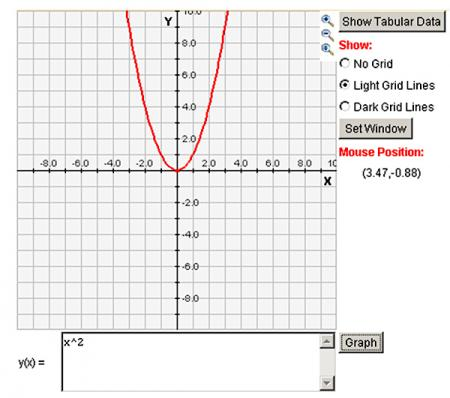# Let's Get Started

Let's explore the different parent functions and learn how to determine if a graph or an equation represents a linear or quadratic function.

TEKS Standards and Student Expectations

A(3) Linear functions, equations, and inequalities. The student applies the mathematical process standards when using graphs of linear functions, key features, and related transformations to represent in multiple ways and solve, with and without technology, equations, inequalities, and systems of equations. The student is expected to:

A(3)(C) graph linear functions on the coordinate plane and identify key features, including
x‐intercept, y‐intercept, zeros, and slope, in mathematical and real world problems

A(7) Quadratic functions and equations. The student applies the mathematical process standards when using graphs of quadratic functions and their related transformations to represent in multiple ways and determine, with and without technology, the solutions to equations. The student is expected to:

A(7)(A) graph quadratic functions on the coordinate plane and use the graph to identify key attributes, if possible, including x‐intercept, y‐intercept, zeros, maximum value, minimum values, vertex, and the equation of the axis of symmetry

Resource Objective(s)

To determine if a graph or verbal description has a linear or quadratic parent function.

Essential Questions

What does a linear function look like as a graph and an equation?

What does a quadratic function look like as a graph and an equation?

Vocabulary

# What Can You See?

Our objective is to learn to recognize the linear and quadratic parent functions given a graph or verbal description.

What is a parent function?
A parent function is the most basic form of a function. It has the same basic properties as others like it, but it has not been moved or stretched in any direction or skewed in any way.

Why do we care?
Parent functions allow us to quickly tell certain traits of their “children.”  For example, my parents have brown eyes, and I have brown eyes. Most people could guess the color of my eyes by meeting my parents.

It is important to remember that you must know whether the traits of a function are related to linear (y = x) or quadratic (y = x2) parent functions.

# Linear Function Lab

You can use an online graphing calculator or the graphing utility applet below to discover information about the linear parent function.

Directions:
1. In the applet below (or on the online site), input a value for x for the equation "y(x) = ____" and click "Graph." This is the linear parent function.

2. Explore the graphs of linear functions by adding or subtracting values to x (such as y(x) = x + 2) or by multiplying x by a constant (such as y(x) = 3x). Remember the linear parent function is y(x) = x.  This is the most basic, simple form of the function.

Directions:

1. Input x2 under the equation editor button "Y=" on the graphing calculator or "y(x) = ____" on the graphing applet link. Click "Graph." This is the quadratic parent function. The image below illustrates what the activity will look like.2. Explore the quadratic parent function and how other quadratic functions can be formed by transforming the parent function. Remember that the quadratic parent function is only y = x2.